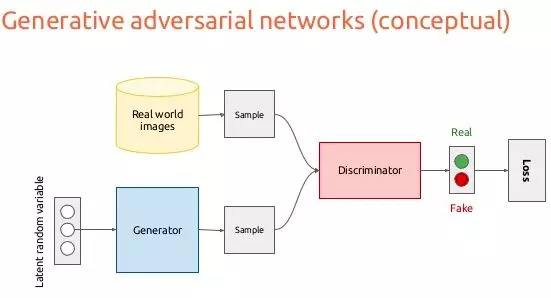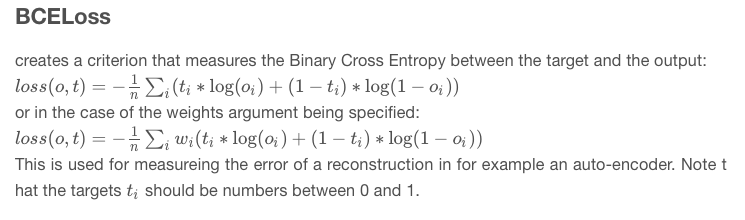# GAN的Loss的比较研究（1）——传统GAN的Loss的理解1

GAN(Generative Adversarial Network)由两个网络组成：Generator网络（生成网络，简称G）、Discriminator网络（判别网络，简称D)，如图：Loss_D只有两个分类，Real image判为1，Fake image（由G生成）判为0，因而可以用二进制交叉熵（BCELoss）来实现Loss_D。

$H(S) = -\sum_i^N P_i\log P_i\qquad(1)$

$H(S)=-\int_x p(x)\log p(x)dx\qquad(2)$

$离散：H(P\vert Q) = -\sum_i^N P_i\log Q_i\qquad(3)\\ 连续：H(P\vert Q)=-\int_x p(x)\log q(x)dx\qquad(4)$
P、Q的顺序不能互换。当P与Q相同时，交叉熵取最小值，此时计算的是P（或Q）的熵。

$H(P|Q)=-( p\log q + (1-p)\log(1- q))\qquad(5)$

$-（1*\log D(x_r)+（1-1)*\log( 1-D(x_r))=-\log D(x_r)$

$-(0*\log D(x_f)+（1-0)*\log( 1-D(x_f))=-\log (1-D(x_f))$

$H(L|D)=-\frac{1}{M}(\sum_{x_r}^M\log (D(x_r))+\sum_{x_f}^M log(1-D(x_f)))\qquad(6)$
D的目标是让$P_d$接近理想概率分布$P_i$$P_i$分布是：real sample输入时，概率输出为1；fake sample输入时，概率输出为0）。因此交叉熵越小越好，即：
$Loss\_D(L, D)=-(\frac{1}{M}\sum_{x_r}^M \log (D(x_r))-\frac{1}{M}\sum_{x_f}^M log(1-D(x_f))\qquad(7)$

（这里解释一下：传统的GAN的object function是：
$\min_G \max_D E_{x\sim q(x)}[\log D(x)]+E_{z\sim p(z)}[\log (1-D(G(z)))]$

$\max_D E_{x\sim q(x)}[\log D(x)]+E_{z\sim p(z)}[\log (1-D(G(z)))]$

$\min_D (-E_{x\sim q(x)}[\log D(x)]-E_{z\sim p(z)}[\log (1-D(G(z)))])$。据此，公式(7)中Loss_D等于目标函数取负号。

$Loss\_D(L, D)= E_{x_r}(-\log (D(x_r)))+ E_{x_f}(-log(1-D(x_f)))\qquad(8)$

y_real_ = torch.ones(mini_batch)    # ground-truth 全为1
y_fake_ = torch.zeros(mini_batch)   # 全为0

x_, y_real_, y_fake_ = Variable(x_.cuda()), Variable(y_real_.cuda()), Variable(y_fake_.cuda())
D_result = D(x_).squeeze()
D_real_loss = BCE_loss(D_result, y_real_)  # 应用BCELoss

z_ = torch.randn((mini_batch, 100)).view(-1, 100, 1, 1)
z_ = Variable(z_.cuda())
G_result = G(z_)

D_result = D(G_result).squeeze()
D_fake_loss = BCE_loss(D_result, y_fake_)  # 应用BCELoss
D_fake_score = D_result.data.mean()

D_train_loss = D_real_loss + D_fake_loss # 实现上述公式7


$Loss\_G=-\log D(x_f)=-(1*\log D(x_f)+0*\log (1-D(x_f))=H(L|D_f)\qquad(9)$
$H(L|D_f)$是输入fake图像，判为1的概率$D_f$，其目标是100%。（9）实际是$D_f$与1的二进制交叉熵。

G_train_loss = BCE_loss(D_result, y_real_) # y_real_全部为1
G_train_loss.backward()
G_optimizer.step()


BCELoss的解释：（在推导的过程中，很容易出现符号的错误。）

GAN很强大，它生成的样本比VAE要更加清晰（边界清楚）和质量更好，但GAN很难训练，体现为两个方面：
1、模型坍塌，即产生的样本单一，没有了多样性。
2、训练过程收敛不易，一方面会出现梯度消失，另一方面会产生巨大的梯度不稳定。
Martin Arjovsky在他那两篇著名文章中，对Loss造成的影响进行了讨论：《Towards principled methods for training generative adversarial networks》、《Wasserstein GAN》。